Support the Monkey! Tell All your Friends and TeachersHome MonkeyNotes Printable Notes Digital Library Study Guides Study Smart Parents Tips College Planning Test Prep Fun Zone Help / FAQ How to Cite Request a New Title

Example In two large populations there are 30% and 25% fair haired people respectively. Is the difference likely to be hidden in sample of 1200 and 900 respectively from the two populations ?

Solution:P1 = 30% = 0.30 and P2 = 25% = 0.25 & q1 = 0.70 and q2 = 0.75 n1 = 1200 and n2 = 900.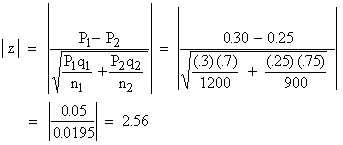Therefore, | z | > 1.96 ( i.e. at 5% level of significance ). Hence it is unlikely that the real difference will be hidden.

Note : At times you may be interested in the comparison of proportions of persons possessing an attribute in a sample with proportion given by the population. In that case use: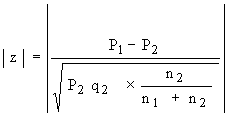where     P2 = Population proportion . q2 = 1 - P2

n1 = Number of observations in the sample

n1 + n2 = Size of population

n2 = (Size of population - n1 )Your browser does not support the IFRAME tag.

Example There are 1000 students in a college out of 20000 students in the whole university. In a study 200 were found smokers in the college and 1000 in the university. Is there a significant difference between the proportion of smokers in the college and in the university?

Solution: Ho : P1 = P2 i.e. there is no significant difference in the college and university in case of proportion of smokers. Ha : P1 ¹ P2.

Proportion of smokers in college P1 =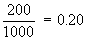Proportion of smokers in the university P2 =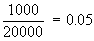q2 = 1 - P2 = 0.95

Also n1 = 1000 and n1 + n2 = 20000. \ n2 = 19000.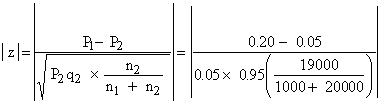Since the value is highly significant, it could not have arisen due to sample fluctuations. Rejecting Ho we say that there is a significant difference between proportion of smokers in the college and the university.

Index

8.1 Population
8.2 Sample
8.3 Parameters and Statistic
8.4 Sampling Distribution
8.5 Sampling Error
8.6 Central Limit Theorem
8.7 Critical Region
8.8 Testing of Hypothesis
8.9 Errors in Tesitng of Hypothesis
8.10 Power o a Hypothesis Test
8.11 Sampling of Variables
8.12 Sampling of Attributes
8.13 Estimation
8.14 Testing the Difference Between Means
8.15 Test for Difference Between Proportions
8.16 Two Tailed and one Tailed Tests
8.17 Test of Significance for Small Samples
8.18 Students t-distribution
8.19 Distribution of 't' for Comparison of Two Samples Means Independent Samples
8.20 Testing Difference Between Mens of Two Samples Dependent Samples or Matched Paired Observations
8.21 Chi-Square
8.22 Sampling Theory of Correlation
8.23 Sampling Theory of Regression

Chapter 1Search: All Products Books Popular Music Classical Music Video DVD Toys & Games Electronics Software Tools & Hardware Outdoor Living Kitchen & Housewares Camera & Photo Cell Phones Keywords: